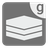Create Intervals (generator version)
Undefined
English RU

From a set of ints you have to create a list of closed intervals as tuples, so the intervals are covering all the values found in the set.
In this mission you should use the 'yield' to make your function a generator.

A closed interval includes its endpoints! The interval 1..5, for example, includes each value x that satifies the condition 1 <= x <= 5.

Values can only be in the same interval if the difference between a value and the next smaller value in the set equals one, otherwise a new interval begins. Of course, the start value of an interval is excluded from this rule.
A single value, that does not fit into an existing interval becomes the start- and endpoint of a new interval.

Input:A set of ints.

Output:A list of tuples of two ints, indicating the endpoints of the interval. The list should be sorted by start point of each interval.

Examples:

```create_intervals({1, 2, 3, 4, 5, 7, 8, 12}) == [(1, 5), (7, 8), (12, 12)]
create_intervals({1, 2, 3, 6, 7, 8, 4, 5}) == [(1, 8)]```